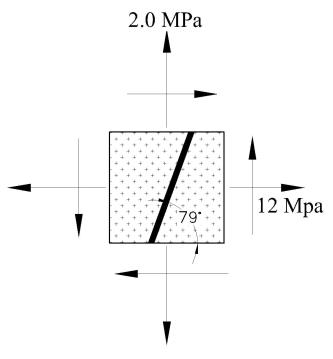# For the state of plane stress shown, determine a) The value of \tau_{xy} for which the in-plane...

## Question:For the state of plane stress shown, determine

a) The value of {eq}\tau_{xy}=\rule{3cm}{0.15mm}{/eq} MPa for which the in-plane shearing stress parallel to the weld is zero.

b) The corresponding principal stresses. {eq}\sigma_a=\rule{3cm}{0.15mm}{/eq} MPa, {eq}\sigma_b=\rule{3cm}{0.15mm}{/eq} MPa.

## Maximum and Minimum Principal Stress:

Maximum principal stress is maximum normal stress built in the body at an angle where shear stress is zero whereas the minimum principal stress is the minimum value of normal stress built in the body at an angle where shear stress is zero.

## Answer and Explanation:

Given Data:

• The normal stress in x direction is: {eq}{\sigma _x} = 12\;{\rm{MPa}} {/eq}
• The normal stress in y direction is: {eq}{\sigma _y} = 2\;{\rm{MPa}} {/eq}
• The plane shearing stress parallel to weld is: {eq}\tau = 0 {/eq}
• The angle of plane from the y face is: {eq}\alpha = 79^\circ {/eq}

(a)

For transformation equation, the oblique angle with respect to x face is,

{eq}\theta = 90 - \alpha {/eq}

Substitute the values in the above equation.

{eq}\begin{align*} \theta &= 90 - 79^\circ \\ &= 11^\circ \end{align*} {/eq}

The formula to calculate shear stress transformation equation is as follows:

{eq}{\tau _s} = - \left( {{\sigma _x} - {\sigma _y}} \right)\sin \theta \cos \theta + {\tau _{xy}}\left( {{{\cos }^2}\theta - {{\sin }^2}\theta } \right) {/eq}

Here, normal stress in x direction is {eq}{\sigma _x} {/eq}, normal stress in the y direction is {eq}{\sigma _y} {/eq} and shear stress in the x-y plane is \${\tau _{xy}